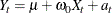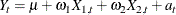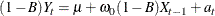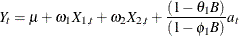The ARIMA Procedure

Input Variables and Regression with ARMA Errors

Subsections:

In addition to past values of the response series and past errors, you can also model the response series using the current and past values of other series, called input series.

Several different names are used to describe ARIMA models with input series. Transfer function model, intervention model, interrupted time series model, regression model with ARMA errors, Box-Tiao model, and ARIMAX model are all different names for ARIMA models with input series. Pankratz (1991) refers to these models as dynamic regression models.

Using Input Series

To use input series, list the input series in a CROSSCORR= option on the IDENTIFY statement and specify how they enter the model with an INPUT= option on the ESTIMATE statement. For example, you might use a series called PRICE to help model SALES, as shown in the following statements:

proc arima data=a;
identify var=sales crosscorr=price;
estimate input=price;
run;

This example performs a simple linear regression of SALES on PRICE; it produces the same results as PROC REG or another SAS regression procedure. The mathematical form of the model estimated by these statements isThe parameter estimates table for this example (using simulated data) is shown in Figure 7.20. The intercept parameter is labeled MU. The regression coefficient for PRICE is labeled NUM1. (See the section Naming of Model Parameters for information about how parameters for input series are named.)

Figure 7.20: Parameter Estimates Table for Regression Model

The ARIMA Procedure

Conditional Least Squares Estimation
Parameter Estimate Standard
Error
t Value Approx
Pr > |t|
Lag Variable Shift
MU 199.83602 2.99463 66.73 <.0001 0 sales 0
NUM1 -9.99299 0.02885 -346.38 <.0001 0 price 0

Any number of input variables can be used in a model. For example, the following statements fit a multiple regression of SALES on PRICE and INCOME:

proc arima data=a;
identify var=sales crosscorr=(price income);
estimate input=(price income);
run;

The mathematical form of the regression model estimated by these statements isLagging and Differencing Input Series

You can also difference and lag the input series. For example, the following statements regress the change in SALES on the change in PRICE lagged by one period. The difference of PRICE is specified with the CROSSCORR= option and the lag of the change in PRICE is specified by the 1 $in the INPUT= option. proc arima data=a; identify var=sales(1) crosscorr=price(1); estimate input=( 1$ price );
run;

These statements estimate the modelRegression with ARMA Errors

You can combine input series with ARMA models for the errors. For example, the following statements regress SALES on INCOME and PRICE but with the error term of the regression model (called the noise series in ARIMA modeling terminology) assumed to be an ARMA(1,1) process.

proc arima data=a;
identify var=sales crosscorr=(price income);
estimate p=1 q=1 input=(price income);
run;

These statements estimate the modelStationarity and Input Series

Note that the requirement of stationarity applies to the noise series. If there are no input variables, the response series (after differencing and minus the mean term) and the noise series are the same. However, if there are inputs, the noise series is the residual after the effect of the inputs is removed.

There is no requirement that the input series be stationary. If the inputs are nonstationary, the response series will be nonstationary, even though the noise process might be stationary.

When nonstationary input series are used, you can fit the input variables first with no ARMA model for the errors and then consider the stationarity of the residuals before identifying an ARMA model for the noise part.

Identifying Regression Models with ARMA Errors

Previous sections described the ARIMA modeling identification process that uses the autocorrelation function plots produced by the IDENTIFY statement. This identification process does not apply when the response series depends on input variables. This is because it is the noise process for which you need to identify an ARIMA model, and when input series are involved the response series adjusted for the mean is no longer an estimate of the noise series.

However, if the input series are independent of the noise series, you can use the residuals from the regression model as an estimate of the noise series, then apply the ARIMA modeling identification process to this residual series. This assumes that the noise process is stationary.

The PLOT option in the ESTIMATE statement produces similar plots for the model residuals as the IDENTIFY statement produces for the response series. The PLOT option prints an autocorrelation function plot, an inverse autocorrelation function plot, and a partial autocorrelation function plot for the residual series. Note that these residual correlation plots are produced by default.

The following statements show how the PLOT option is used to identify the ARMA(1,1) model for the noise process used in the preceding example of regression with ARMA errors:

proc arima data=a;
identify var=sales crosscorr=(price income) noprint;
estimate input=(price income) plot;
run;
estimate p=1 q=1 input=(price income);
run;

In this example, the IDENTIFY statement includes the NOPRINT option since the autocorrelation plots for the response series are not useful when you know that the response series depends on input series.

The first ESTIMATE statement fits the regression model with no model for the noise process. The PLOT option produces plots of the autocorrelation function, inverse autocorrelation function, and partial autocorrelation function for the residual series of the regression on PRICE and INCOME.

By examining the PLOT option output for the residual series, you verify that the residual series is stationary and identify an ARMA(1,1) model for the noise process. The second ESTIMATE statement fits the final model.

Although this discussion addresses regression models, the same remarks apply to identifying an ARIMA model for the noise process in models that include input series with complex transfer functions.